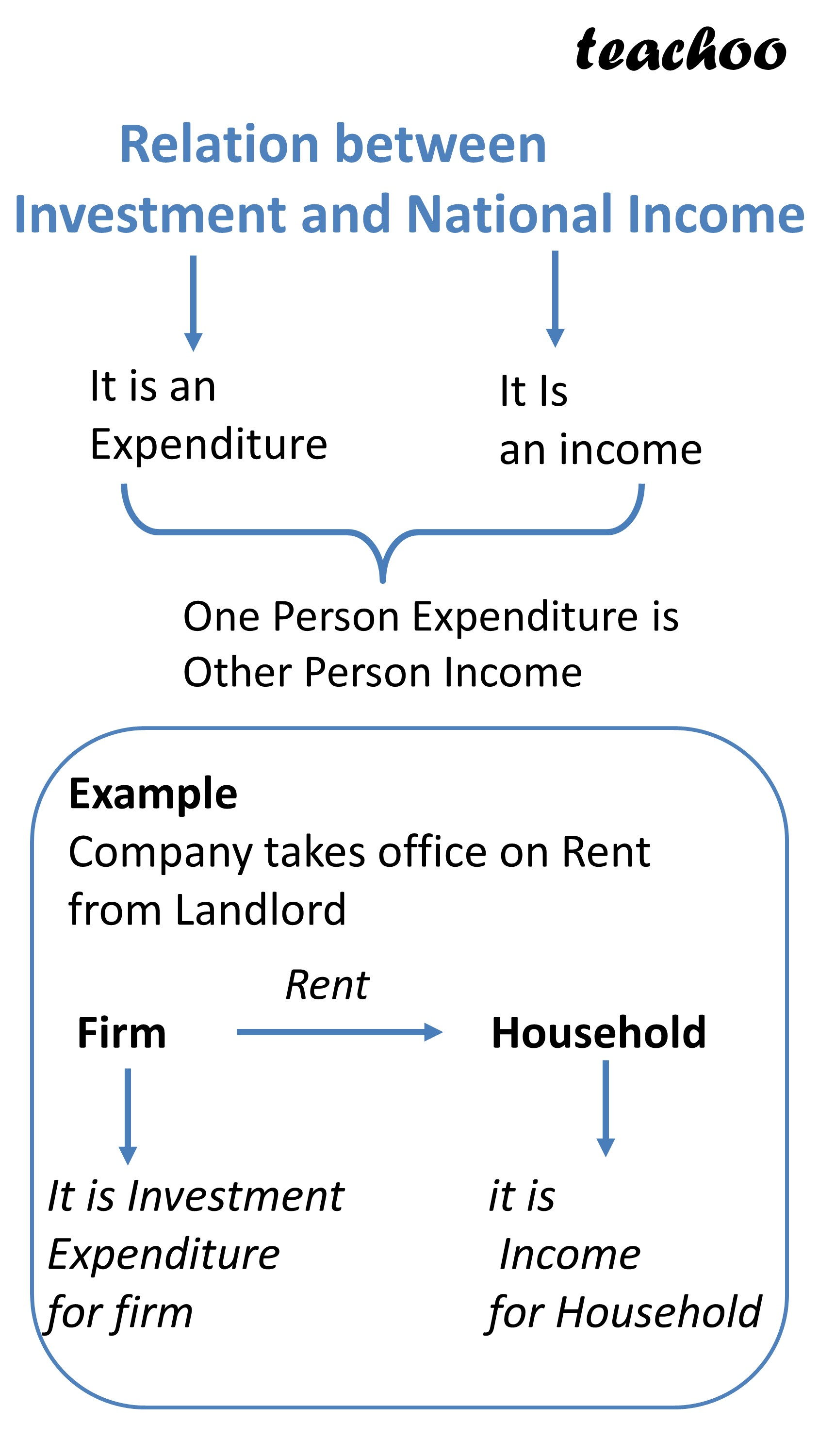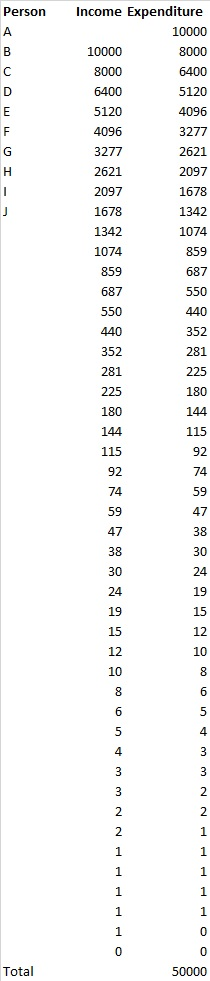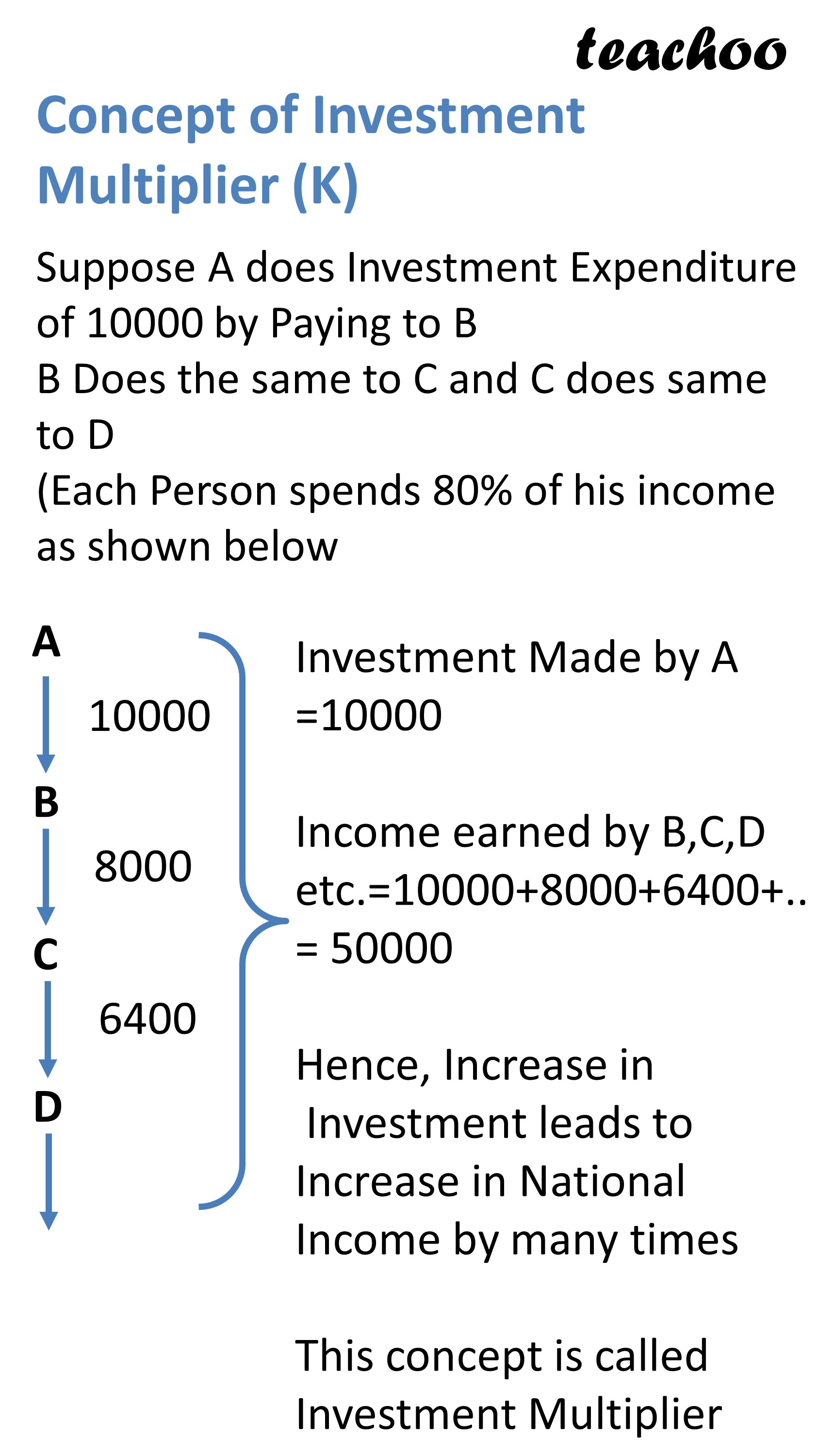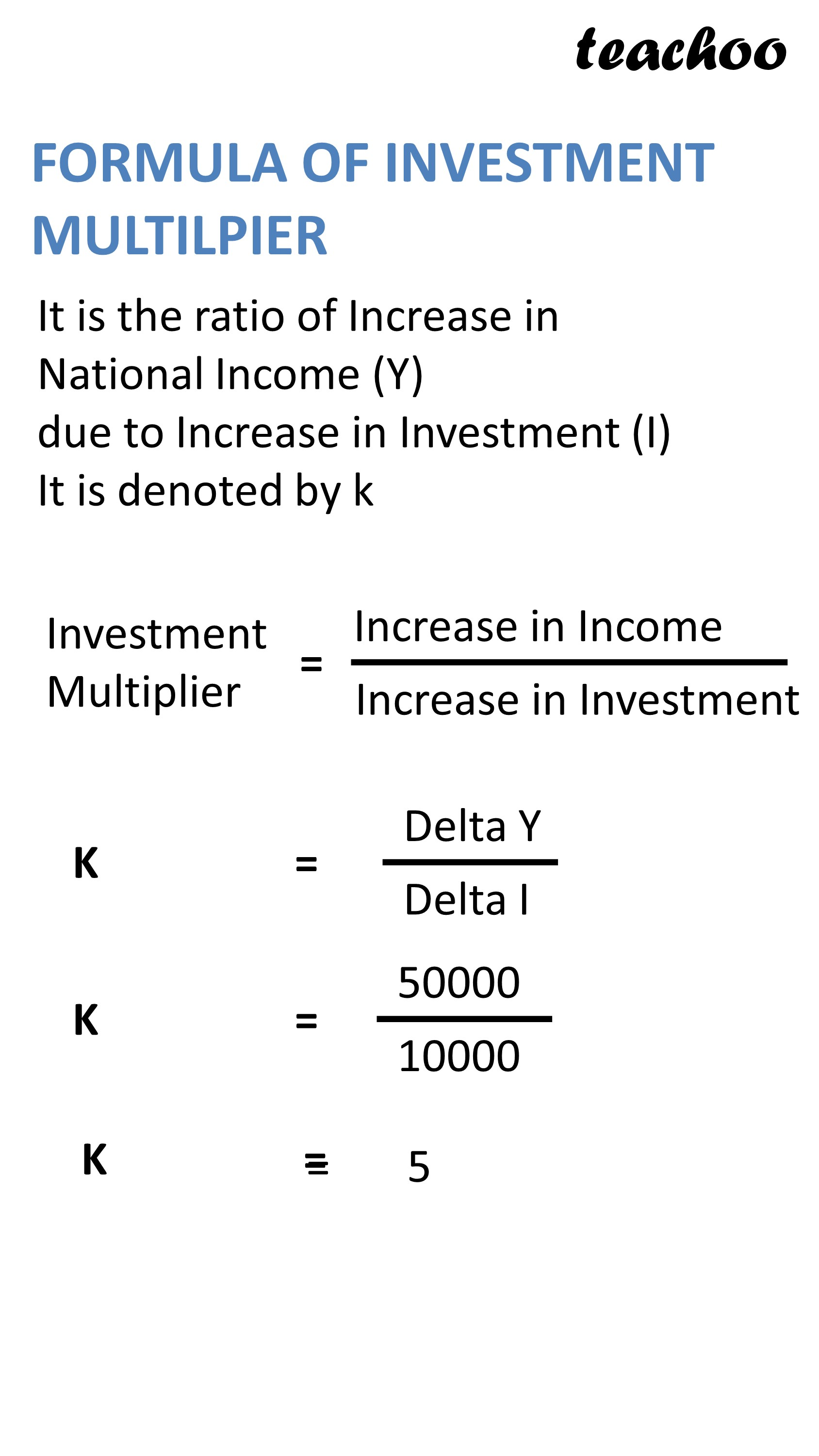Chapter 4 Part 2 - Income Determination and Multiplier

Economics Class 12
Macroeconomics

## How is Investment and National Income linked?

We know that

Investment is an Expenditure

This expenditure of one person is income of other person and the process continues

hence, Investment and Income are linked### Example 1

Suppose there are only 2 persons in economy- F (Firm) and Household (H)

Suppose Firm Increases its Investment Expenditure by 10000

It will do so by paying salary /rent etc to H of Rs 10000

This 10000 will become Income of H

Hence, Increase in Investment of F lead to increase in Income of Household

### Example 2

A incurred Investment Expenditure of Rs 10000 by paying wages to B

This Rs 10000 is now income of B

Suppose Marginal Propensity to consume is 80%

It means B will spend 8000 out of this 10000 by paying to C

Hence, this 80000 becomes income of C

Suppose C spends 6400 out of this 8000 by paying to D

Hence, this 6400 becomes income of D and this process continues as underIn this case,

Income earned by B,C,D etc=10000+8000+6400+..=50000

Hence, Increase in Investment leads to Increase in National Income by many times

This concept is called Investment Multiplier## What is Investment Multiplier?

It is the ratio of Increase in National Income(Y) due to Increase in Investment (I)

It is denoted by k

Investment Multiplier=Increase In Income / Increase in Investment

K = Delta Y / Delta IExample 1

Suppose on investment of 50000 ,Income earned is 10000

If investment increased to 60000,Income becomes 30000

In this case

Increase in Income =30000-10000=20000

Delta Y=20000

Increase in Investment =60000-50000=10000

Delta I=10000

Investment Multiplier=Increase In Income / Increase in Investment

K = Delta Y / Delta I

K = 20000 / 10000

K = 2

Example 2

An additional Investment of 20000 Crore in economy generate additional income of 30000 Cr

What is Value of Investment Multiplier?

Investment Multiplier=Increase In Income / Increase in Investment

K = Delta Y / Delta I

K = 30000 / 20000

K = 1.5

Learn in your speed, with individual attention - Teachoo Maths 1-on-1 Class

### Transcript

Relation between Investment and National Income It is an Expenditure It Is an income One Person Expenditure is Other Person Income Example Company takes office on Rent from Landlord It is Investment Expenditure for firm it is Income for Household Concept of Investment Multiplier (K) Suppose A does Investment Expenditure of 10000 by Paying to B B Does the same to C and C does same to D (Each Person spends 80% of his income as shown below 10000 8000 6400 Investment Made by A =10000 Income earned by B,C,D etc.=10000+8000+6400+.. = 50000 Hence, Increase in Investment leads to Increase in National Income by many times This concept is called Investment Multiplier FORMULA OF INVESTMENT MULTILPIER It is the ratio of Increase in National Income (Y) due to Increase in Investment (I) It is denoted by k Increase in Investment